• 一 高斯朴素贝叶斯分类器代码实现 网上搜索不调用sklearn实现的朴素贝叶斯分类器基本很少，即使有也是结合文本分类的多项式或伯努利类型，因此自己写了一遍能直接封装的高斯类型NB分类器，当然与真正的源码相比少了...

一 高斯朴素贝叶斯分类器代码实现
网上搜索不调用sklearn实现的朴素贝叶斯分类器基本很少，即使有也是结合文本分类的多项式或伯努利类型，因此自己写了一遍能直接封装的高斯类型NB分类器，当然与真正的源码相比少了很多属性和方法，有兴趣的可以自己添加。代码如下（有详细注释）：
class NaiveBayes():
'''高斯朴素贝叶斯分类器'''

def __init__(self):

self._X_train = None
self._y_train = None
self._classes = None
self._priorlist = None
self._meanmat = None
self._varmat = None

def fit(self, X_train, y_train):

self._X_train = X_train
self._y_train = y_train
self._classes = np.unique(self._y_train)                       #  得到各个类别
priorlist = []
meanmat0 = np.array([[0, 0, 0, 0]])
varmat0 = np.array([[0, 0, 0, 0]])
for i, c in enumerate(self._classes):
# 计算每个种类的平均值，方差，先验概率
X_Index_c = self._X_train[np.where(self._y_train == c)]        # 属于某个类别的样本组成的“矩阵”
priorlist.append(X_Index_c.shape / self._X_train.shape)  # 计算类别的先验概率
X_index_c_mean = np.mean(X_Index_c, axis=0, keepdims=True)     # 计算该类别下每个特征的均值，结果保持二维状态[[3 4 6 2 1]]
X_index_c_var = np.var(X_Index_c, axis=0, keepdims=True)       # 方差
meanmat0 = np.append(meanmat0, X_index_c_mean, axis=0)         # 各个类别下的特征均值矩阵罗成新的矩阵，每行代表一个类别。
varmat0 = np.append(varmat0, X_index_c_var, axis=0)
self._priorlist = priorlist
self._meanmat = meanmat0[1:, :]                                    #除去开始多余的第一行
self._varmat = varmat0[1:, :]

def predict(self,X_test):

eps = 1e-10                                                        # 防止分母为0
classof_X_test = []                                                #用于存放测试集中各个实例的所属类别
for x_sample in X_test:
matx_sample = np.tile(x_sample,(len(self._classes),1))         #将每个实例沿列拉长，行数为样本的类别数
mat_numerator = np.exp(-(matx_sample - self._meanmat) ** 2 / (2 * self._varmat + eps))
mat_denominator = np.sqrt(2 * np.pi * self._varmat + eps)
list_log = np.sum(np.log(mat_numerator/mat_denominator),axis=1)# 每个类别下的类条件概率取对数后相加
prior_class_x = list_log + np.log(self._priorlist)             # 加上类先验概率的对数
prior_class_x_index = np.argmax(prior_class_x)                 # 取对数概率最大的索引
classof_x = self._classes[prior_class_x_index]                 # 返回一个实例对应的类别
classof_X_test.append(classof_x)
return classof_X_test

def score(self, X_test, y_test):

j = 0
for i in range(len(self.predict(X_test))):
if self.predict(X_test)[i] == y_test[i]:
j += 1
return ('accuracy: {:.10%}'.format(j / len(y_test)))
对于手动实现的高斯型NB分类器，利用鸢尾花数据进行测试，与调用sklearn库的分类器结果差不多，基本在93-96徘徊。这是由于多次进行二八切分，相当于多次留出法。为计算更准确精度，可进行交叉验证并选择多个评价方法，这里不再实现。
import numpy as np
from sklearn import datasets
from sklearn.model_selection import train_test_split
from sklearn import preprocessing
# 获取数据集，并进行8:2切分
X = iris.data
y = iris.target
# print(X)
X_train, X_test, y_train, y_test = train_test_split(X, y, test_size=0.2)

nb = NaiveBayes()
nb.fit(X_train,y_train)
print(nb.predict(X_test))
print(nb.score(X_test,y_test))
#输出结果如下：
[0, 2, 1, 1, 1, 2, 1, 0, 2, 0, 1, 1, 1, 0, 2, 2, 2, 2, 0, 1, 1, 0, 2, 2, 2, 0, 1, 0, 1, 0]
accuracy: 96.6666666667%
二 其他
基于属性条件独立性假设的朴素贝叶斯，在现实中往往很难成立，因此产生了“半朴素贝叶斯分类器”。其基本思想是适当考虑一部分属性间的相互依赖信息，从而既不需要进行完全联合概率计算，又不至于彻底忽略比较强的属性依赖关系。“独依赖估计”是最常用的一种策略，即假设每个属性在类别之外最多依赖一个其他属性。包括SPODE方法，TAN方法，AODE方法等。
np.unique():返回原来array中不重复元素组成的新array，元素从小到大。
y = np.array([1, 2, 9, 1,2,3])
classes = np.unique(y)                     # 返回y中所有不重复的元素组成的新array([1,2,3,9])
print(classes)                             # 结果为np.array([1,2,3,9])
np.where():对array进行操作
'''
1. np.where(condition, x, y)
满足条件(condition)，满足进行x操作，不满足进行y操作
'''
a= np.array([[9, 7, 3], [4, 5, 2], [6, 3, 8]])
b=np.where(a > 5, 1, 0)               #对于a中的元素如果大于5，则改写成1，否则写成0.
print(b)
输出结果：
[[1 1 0]
[0 0 0]
[1 0 1]]
'''
2. np.where(condition)
只有条件 (condition)，没有x和y，则输出满足条件元素的坐标 (等价于numpy.nonzero)。
这里的坐标以tuple的形式给出，通常原数组有多少维，输出的tuple中就包含几个数组，分别对应符合条件元素的各维坐标。
'''
c = np.array([[9, 7, 3], [4, 5, 2], [6, 3, 8]])
d = np.where(c > 5)                                                #条件为元素大于5
print(d)
输出结果如下（元组）：
(array([0, 0, 2, 2], dtype=int64), array([0, 1, 0, 2], dtype=int64)) 表示下表为 00,01 20,22的元素满足条件。

a = np.array([1,3,6,9,0])
b = np.where(a > 5)
print(b)
输出结果(array([2, 3], dtype=int64),)表示坐标为2和3的元素满足，注意末尾的逗号，表明一维时实质输出元组为二维，2_,3_只不过后面没有而已，a维数大于等于2时，元组和a维数相同。
输出的结果是可以直接作为数组下标。
x = np.array([[1, 5, 8, 1], [2, 4, 6, 8], [3, 6, 7, 9], [6, 8, 3, 1]])
print(x[b])  结果为x的第2,3行组成的数组[[3  6 7 9]  [6  8 3 1]],等价于x[[2,3]],x[2,3]为输出为元素9，x[,]输出数组。

转载于:https://www.cnblogs.com/lyxML/p/9526089.html
展开全文• 贝叶斯分类器理论知识。包括贝叶斯决策论，朴素贝叶斯分类器，半朴素贝叶斯分类器
学习贝叶斯分类器，理论。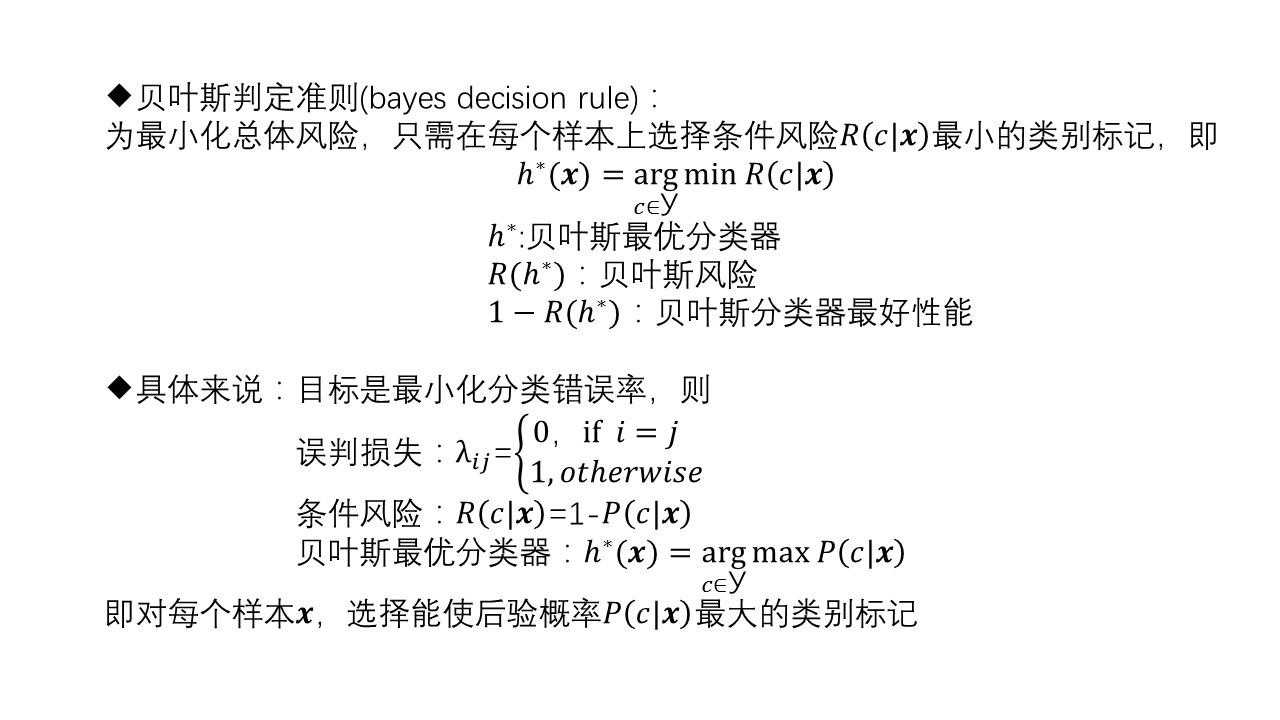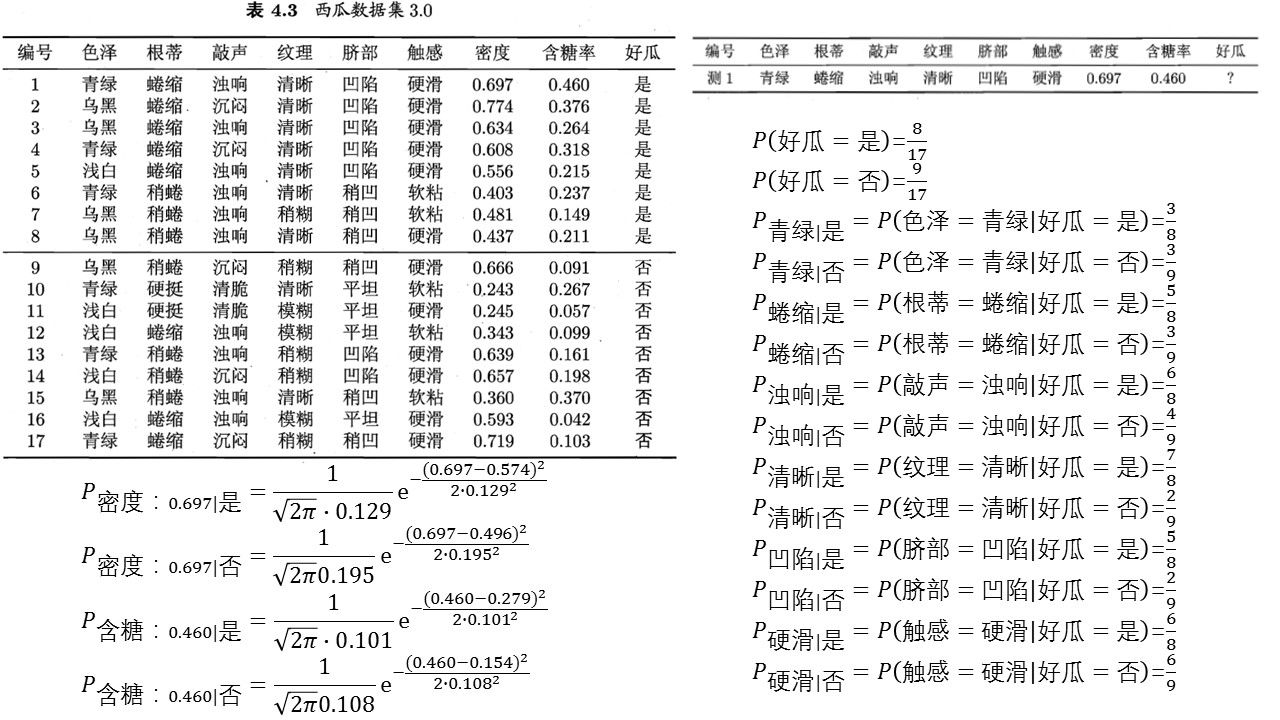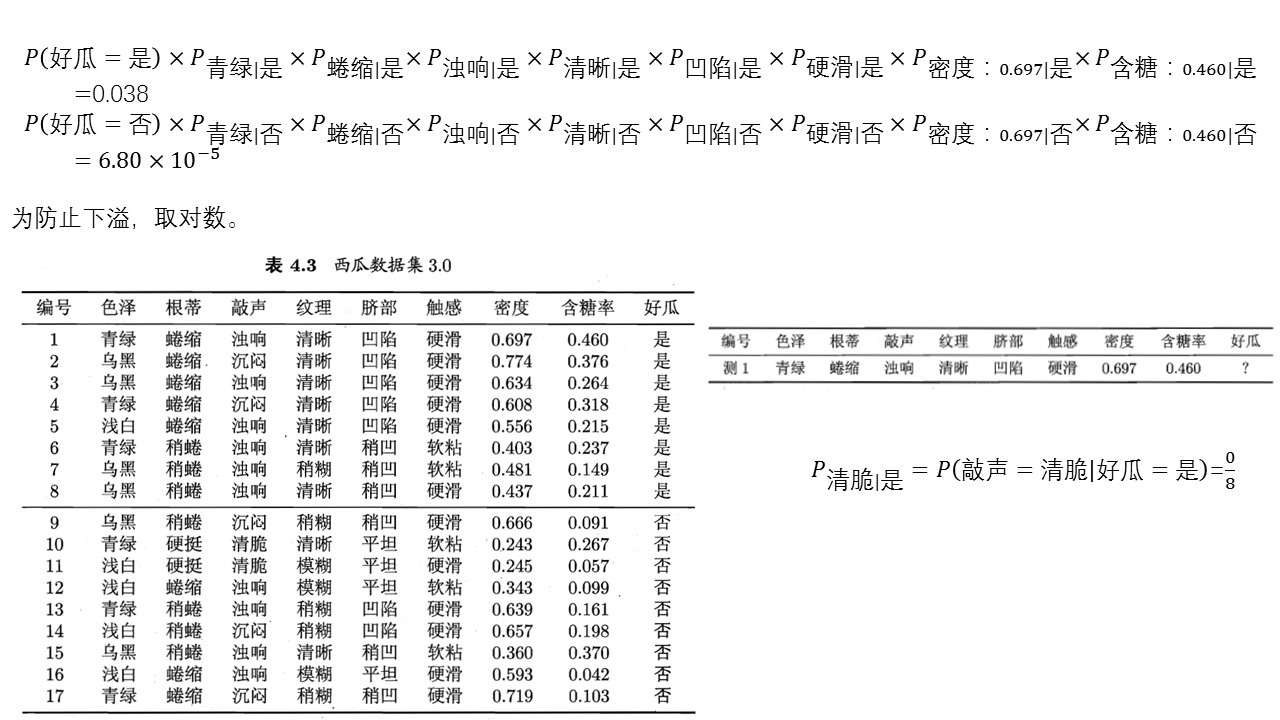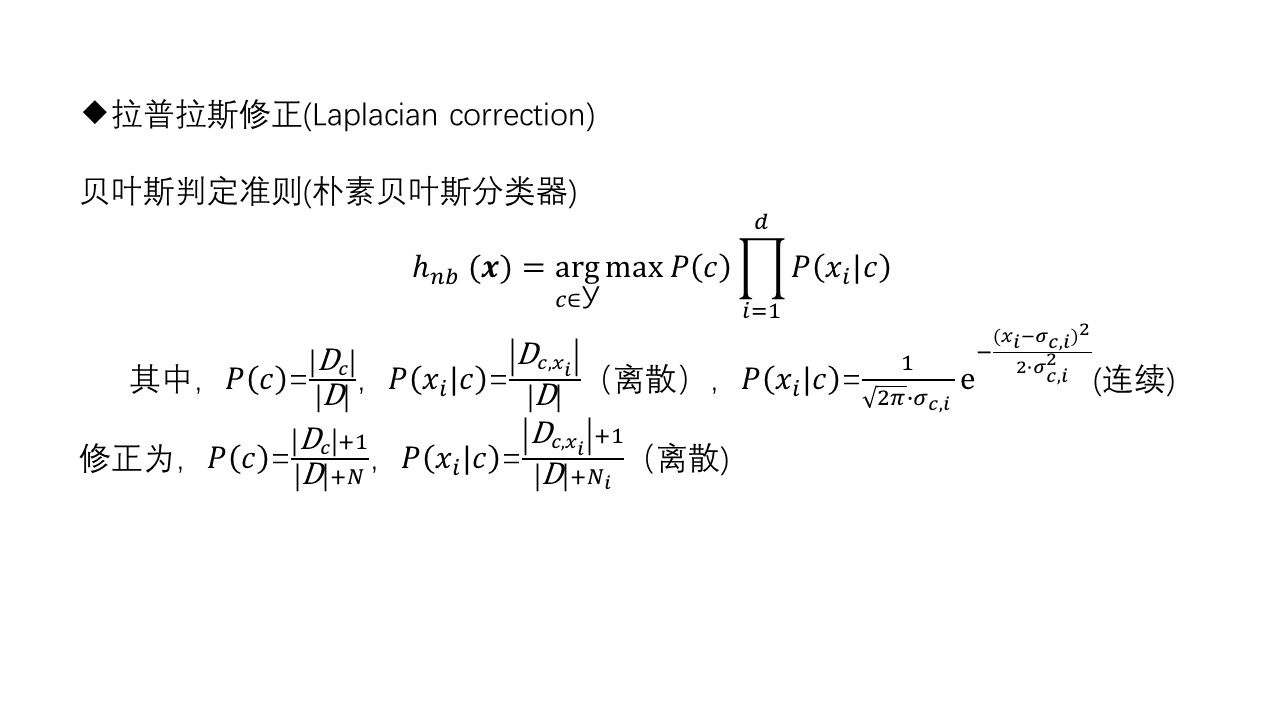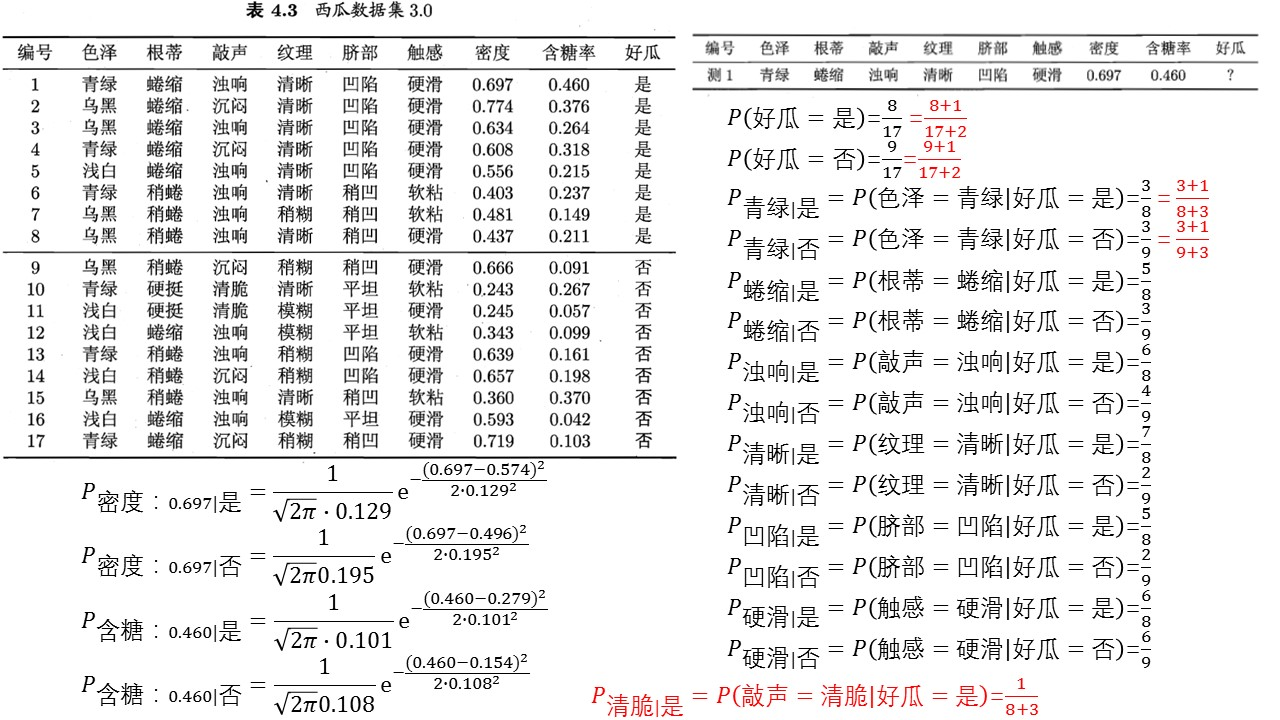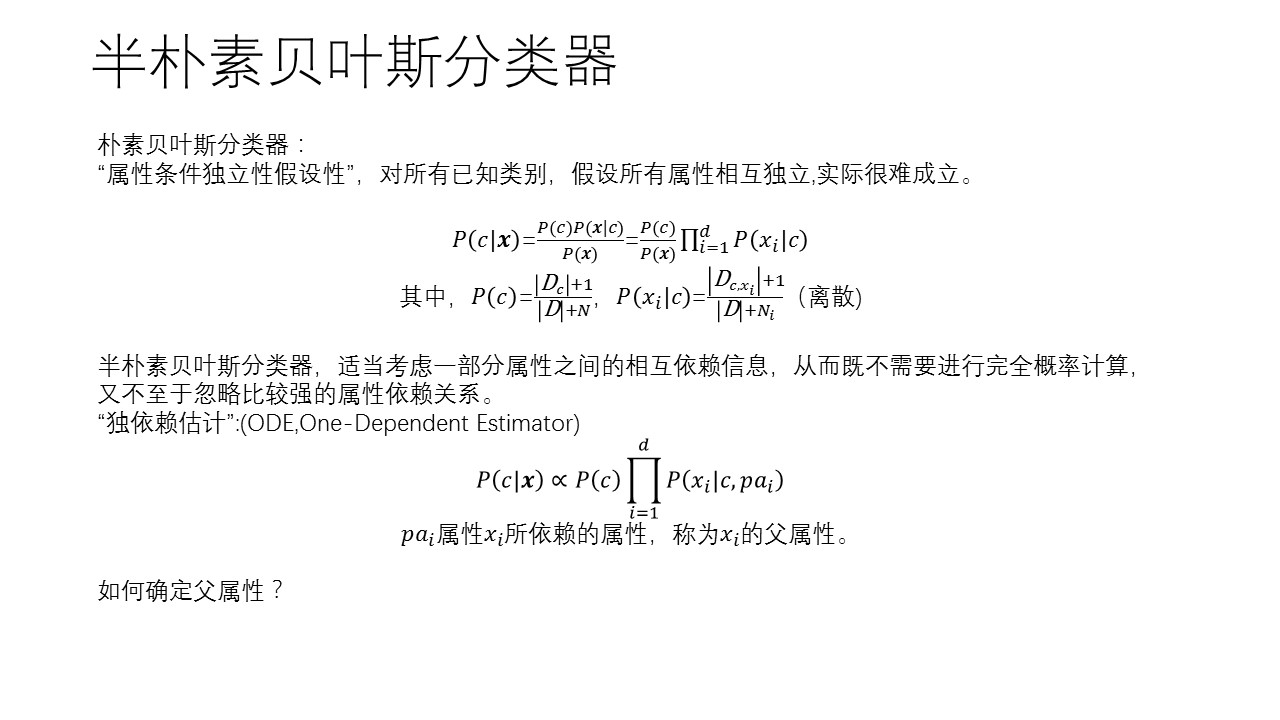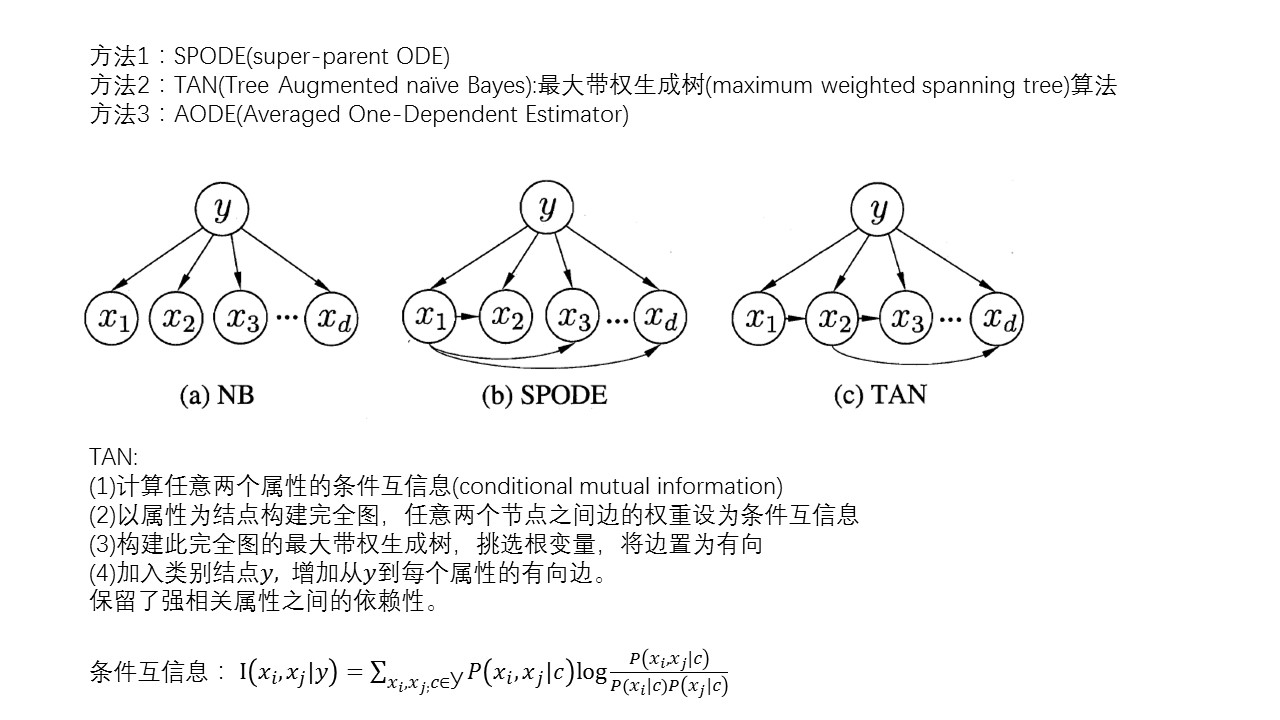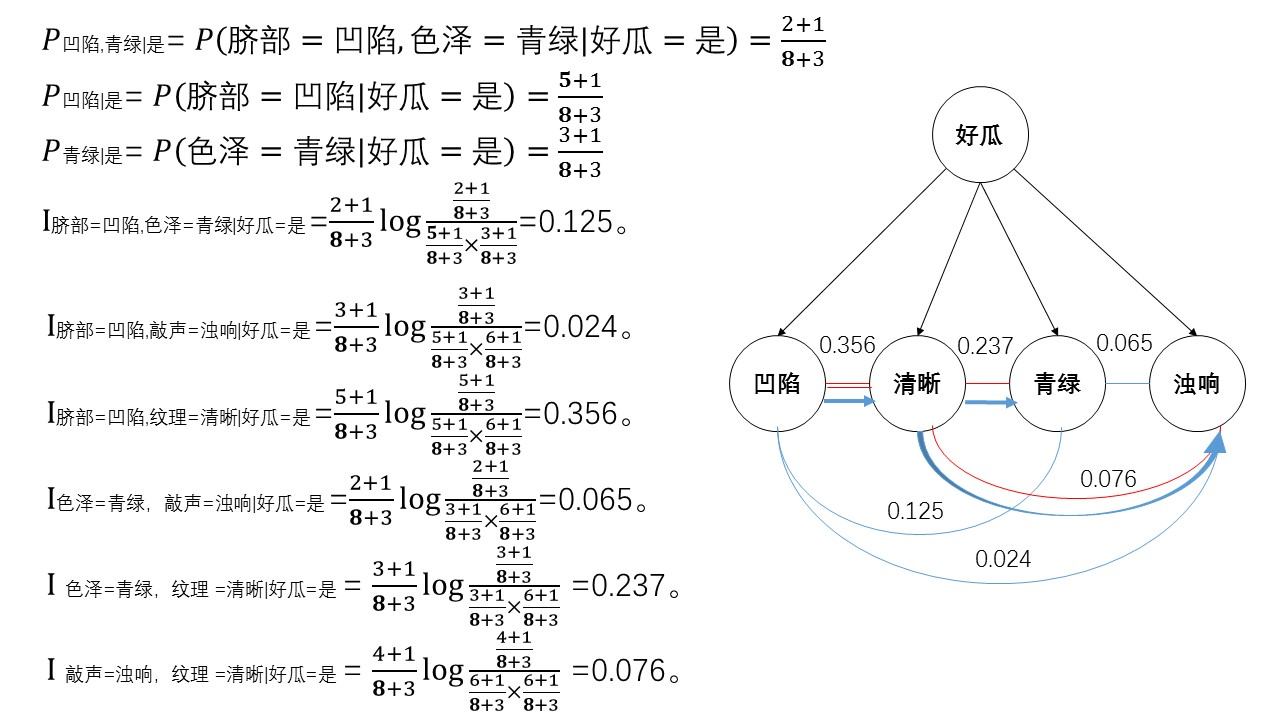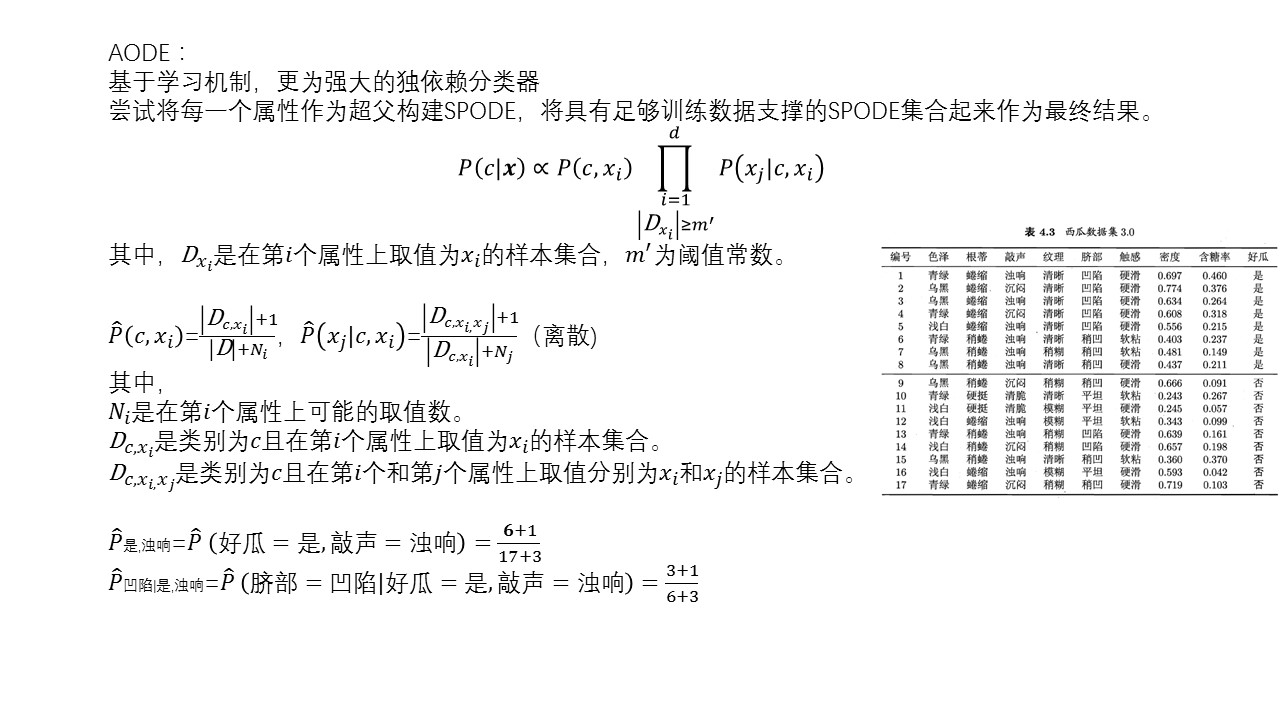展开全文贝叶斯分类器
• 朴素贝叶斯（Naive Bayes）原理+编程实现拉普拉斯修正的朴素贝叶斯分类器，以西瓜数据集3.0为训练集，对“测1”样本进行判别。
贝叶斯方法与朴素贝叶斯1.生成模型与判别模型2.贝叶斯2.1贝叶斯公式2.2贝叶斯方法3朴素贝叶斯3.1条件独立性假设3.2朴素贝叶斯Naive在何处？3.3朴素贝叶斯的三种模型3.4平滑技术3.5朴素贝叶斯优缺点3.6朴素贝叶斯的应用与代码实现
1.生成模型与判别模型
在概率生成模型(Probabilistic Generative Model)与朴素贝叶斯(Naive Bayes)中大概学习了一下概率生成模型以及朴素贝叶斯，今天在这里再总结一下生成模型与判别模型。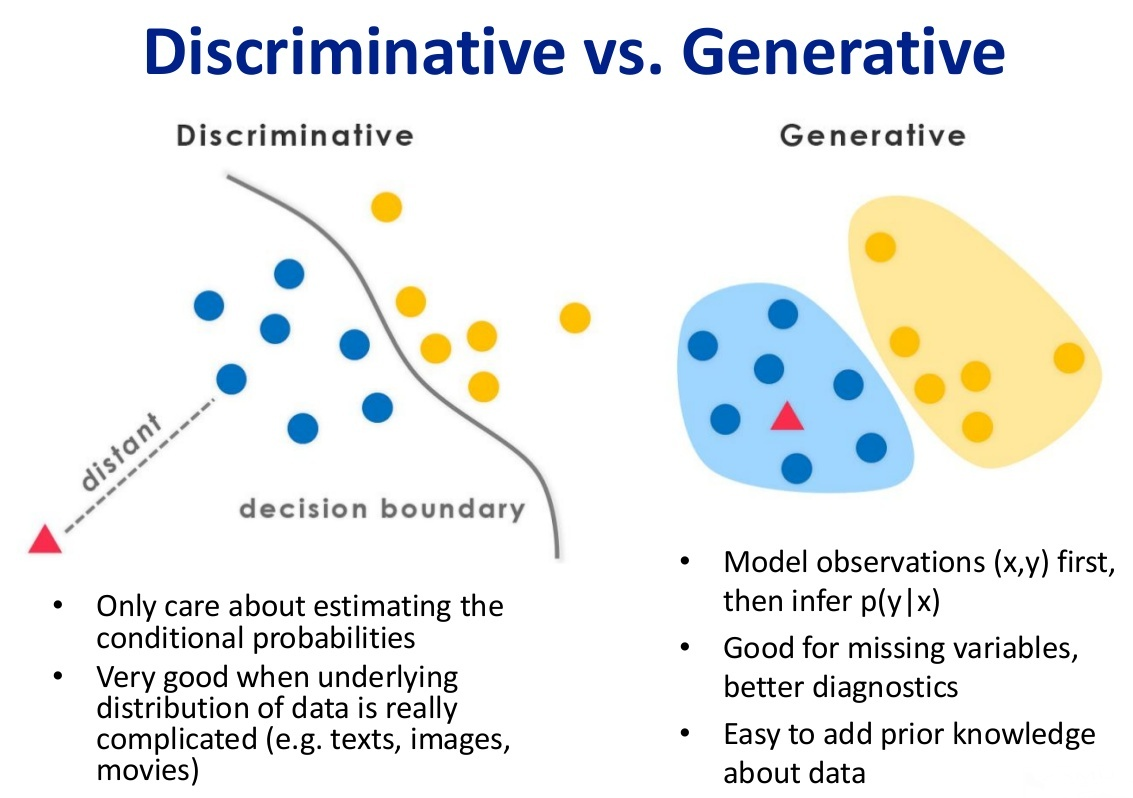上面这张图可以清楚的解释二者的差别。前面我们讲到， 所谓的机器学习模型，本质上是一个函数，其作用是实现从一个样本 $x$ 到样本的标记值 $y$ 的映射，即 $f(x;\theta ^{*})\rightarrow y$，或者求得标签的条件概率：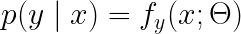例如有C1以及C2两种类别，我们需要判断样本属于哪一个class：

判别式模型：要确定一个样本是属于C1还是C2，用判别模型的方法是从历史数据中学习到模型，然后通过提取该样本的特征来预测出该样本属于C1的概率以及C2的概率。
生成式模型：根据C1的特征首先学习出一个C1的模型，然后根据C2的特征学习出一个C2的模型，然后从该样本中提取特征，放到C1模型中看概率是多少，然后再放到C2模型中看概率是多少，哪个大就是哪个。
判别模型之所以称为判别模型，就是因为它只是简单地判别就好了。根据样本X来判别它所属的类别，直接就求出了$p(y|x)$的大小
而生成模型，在概率生成模型(Probabilistic Generative Model)与朴素贝叶斯(Naive Bayes)中我们可以看到，我们要求：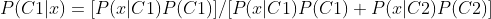P(C1)与P(C2)很好求，我们要求的是P(x|C1)以及P(x|C2)，也就是说我们要先求得C1与C2的具体分布，也就是上面说的，分别根据C1与C2的特征学得两个模型，得到模型之后代入，若P(C1|x)>0.5那x就属于C1，否则属于C2。

2.贝叶斯
2.1贝叶斯公式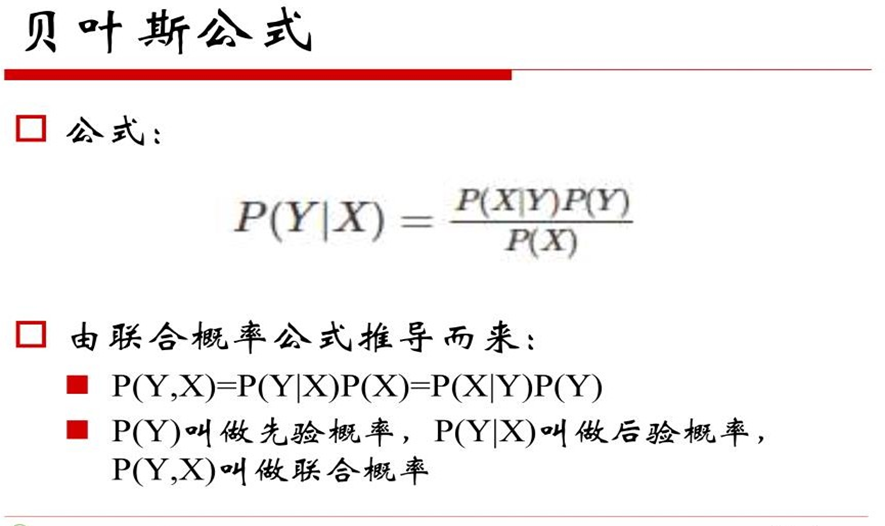学过概率论的都应该对上面这个公式很熟悉，这里就不再细讲了。这里需要注意的点是注意区分先验概率、后验概率、联合概率三个概念。
2.2贝叶斯方法
机器学习的最终目的就是回归 or 分类，这里二者都可以理解为预测，回归很好理解，分类也可以理解为预测属于某一类的概率是多少。 我们把上述贝叶斯公式中的X理解为“具有某特征”，把Y理解成“类别标签”，那么贝叶斯公式就可以表示为：
P(“属于某类”|“具有某特征”)=P(“具有某特征”|“属于某类”)P(“属于某类”)/P(“具有某特征”)。
贝叶斯方法把计算“具有某特征条件下属于某类（就是分类）”的概率转化为需要计算“属于某类条件下具有某特征（分别训练模型）”的概率，属于有监督学习。
3朴素贝叶斯
我们以垃圾邮件识别来引出朴素贝叶斯。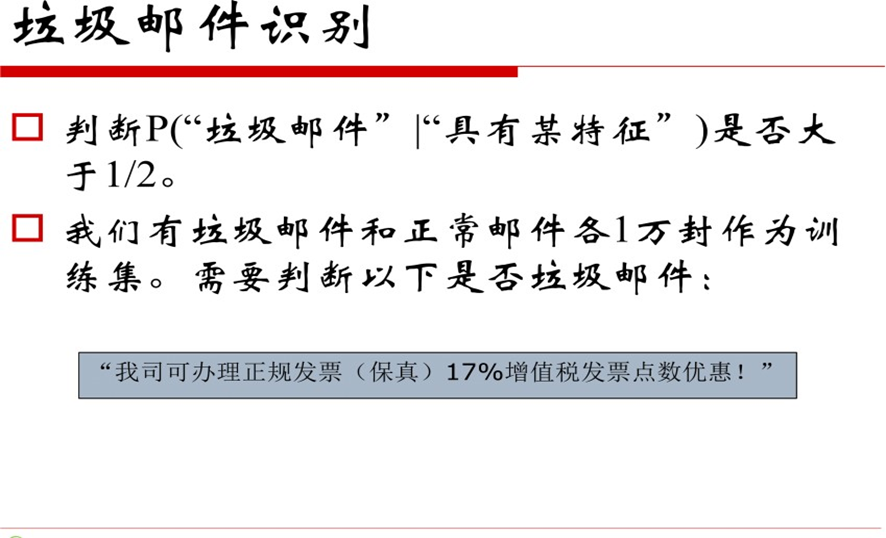我们要做的是判断上面那个邮件：“我司可办理正规发票（保真）17%增值税发票点数优惠”是否属于垃圾邮件。我们不能直接判断一整个邮件是否属于垃圾邮件，我们首先想到的应该是“分词”，所谓分词就是把邮件拆成一个个的词语，通过该词语是否属于训练样本中的垃圾邮件来做出进一步判断：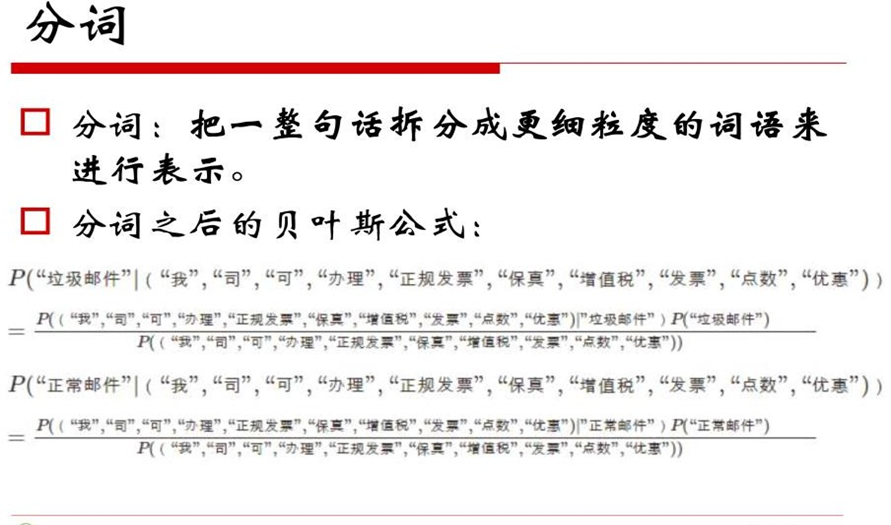前面我们提到：
贝叶斯方法把计算“具有某特征条件下属于某类（就是分类）”的概率转化为需要计算“属于某类条件下具有某特征（分别训练模型）”的概率，属于有监督学习。 也就是说，我们现在要计算的是：正常邮件 or 垃圾邮件中具有上述那些词语的概率。
3.1条件独立性假设
引入条件独立假设：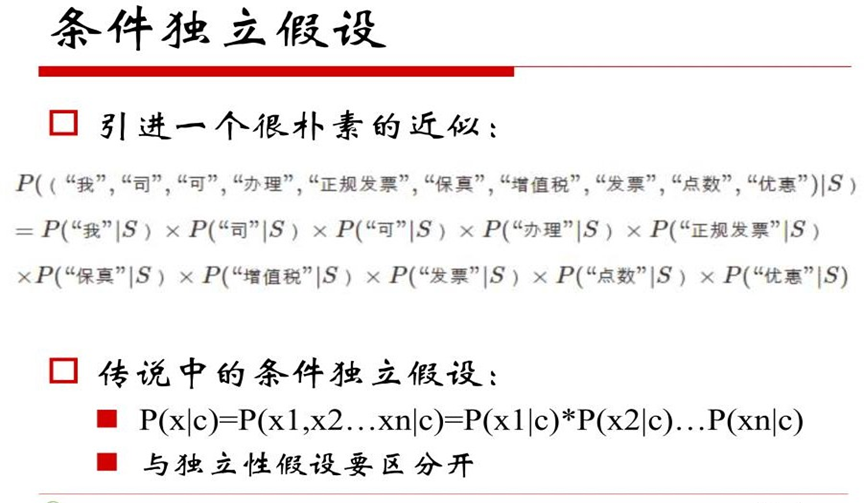我们把求某一类邮件中包含上述那些词语的概率等同于某一类邮件中包含每一种词语概率的乘积！！这其实就是朴素贝叶斯的实质，也是条件独立假设的实质。
既然如此，那每一项都变得特别好求解了：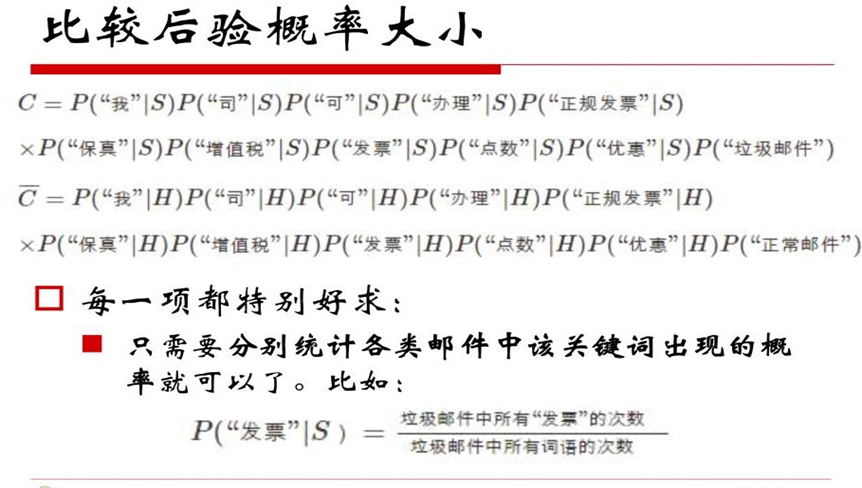要算P(x1|C)，那就直接在训练集中统计一下，看看x1在类别c1中出现的概率是多少即可。
3.2朴素贝叶斯Naive在何处？

加上条件独立假设的贝叶斯方法就是朴素贝叶斯方法（Naive Bayes）
由于乘法交换律，朴素贝叶斯中算出来交换词语顺序的条件概率完全一样

上述2的意思是：对于朴素贝叶斯模型来讲，“我司可办理正规发票”与“正规发票可办理我司”是一样的，会给出相同的判别结果，这点应该很好理解，因为你有了条件独立假设，abc与cba肯定大小是一样的，自然概率也是一样的，判别结果也就一样了。
3.3朴素贝叶斯的三种模型

多项式模型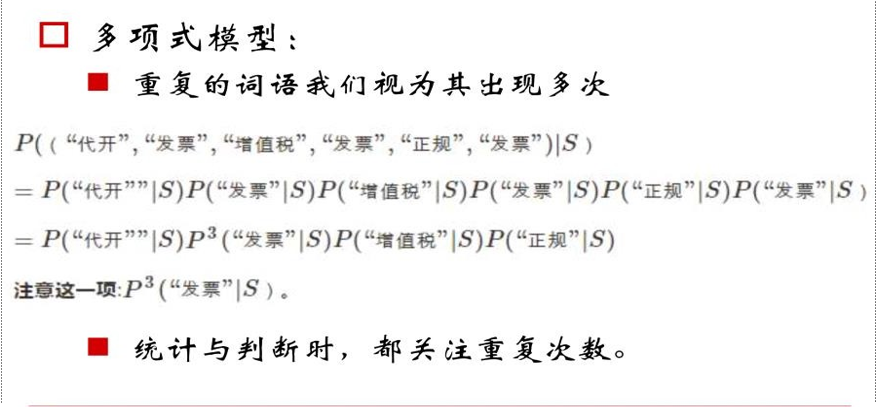多项式模型跟我们思维是一样的，例如在上述条件概率中，“发票”一词语出现了三次，那利用条件独立假设条件之后，自然应该出现三次方，这就是多项式模型。

伯努利模型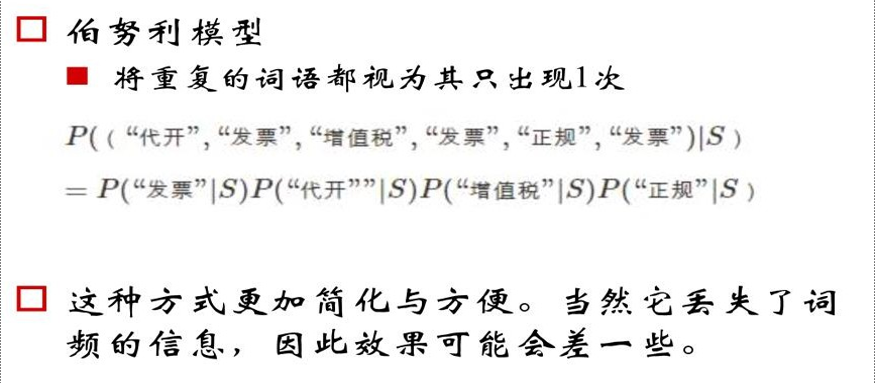在伯努利模型中我们可以看到，即使“发票”一词出现三次，但是我们在最终计算时只算一次。

混合模型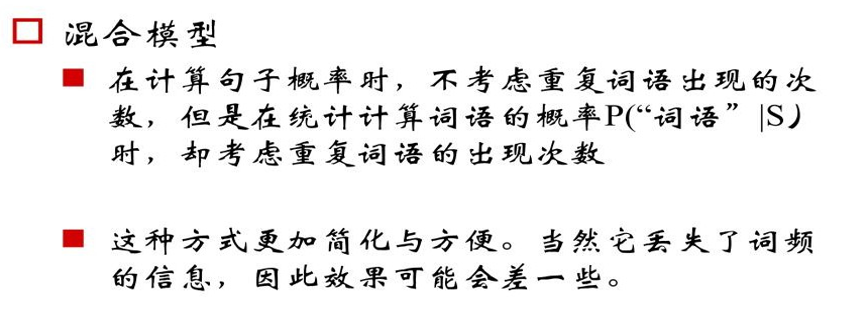在混合模型中，我们计算句子概率时不考虑重复词语出现的次数，但在统计计算词语概率时考虑重复次数。
一张图概括三种模型：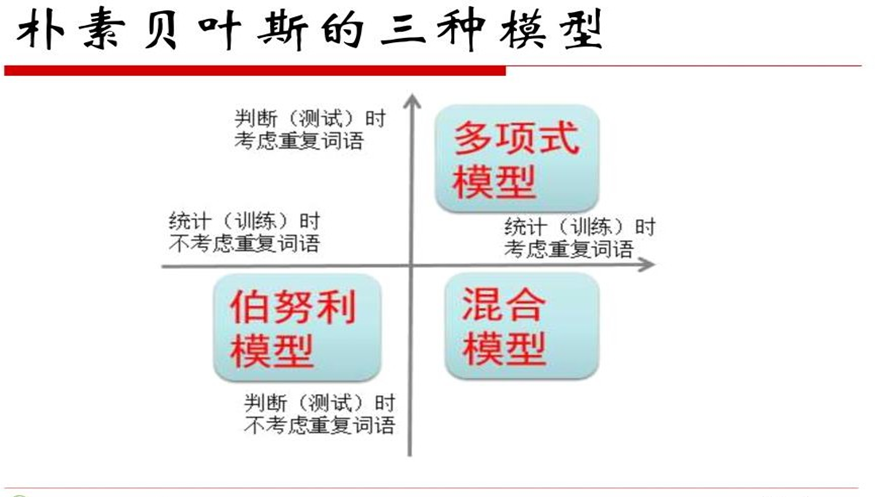3.4平滑技术
我们先来看这样一种可能：我们在求P(“正规发票”|S)时，通常的做法就是在训练集中遍历，看看“正规发票”一词出现了多少次，但是好巧不巧，该词在训练集中一次都没有出现过，那概率不就是0了么，然后条件独立假设相乘。。。于是整个概率都变成了0，这显然是极不合理的，但是这种情况却不少见。
于是我们引入了平滑技术这一概念来解决这个问题：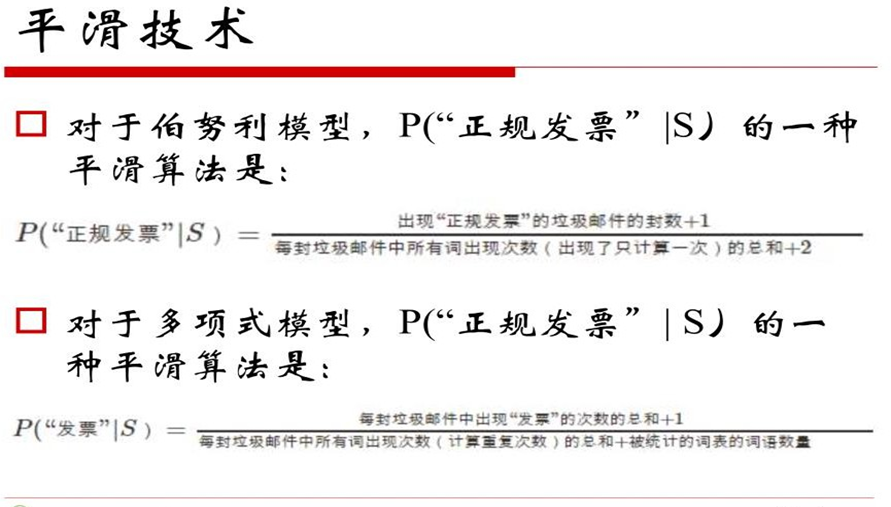这样，上述概率就不为0了，方便后续判断。
3.5朴素贝叶斯优缺点
优点：

对待预测样本进行预测，过程简单速度快
对于多分类问题也同样有效，复杂度也不会有大程度地上升。
在分布独立这个假设成立的情况下，贝叶斯的分类效果很好，会略胜于逻辑回归，我们需要的样本量也更少一点。
对于类别类的输入特征变量，效果非常好。对于数值型变量特征，我们默认它符合正态分布。

缺点：

如果测试集中的一个类别变量特征在训练集里面没有出现过，那么概率就是0，预测功能就将失效，平滑技术可以解决这个问题
朴素贝叶斯中有分布独立的假设前提，但是在现实生活中，这个条件很难满足。

3.6朴素贝叶斯的应用与代码实现
作业：编程实现拉普拉斯修正的朴素贝叶斯分类器，并以西瓜数据集3.0为训练集，对“测1”样本进行判别。
数据集长这样：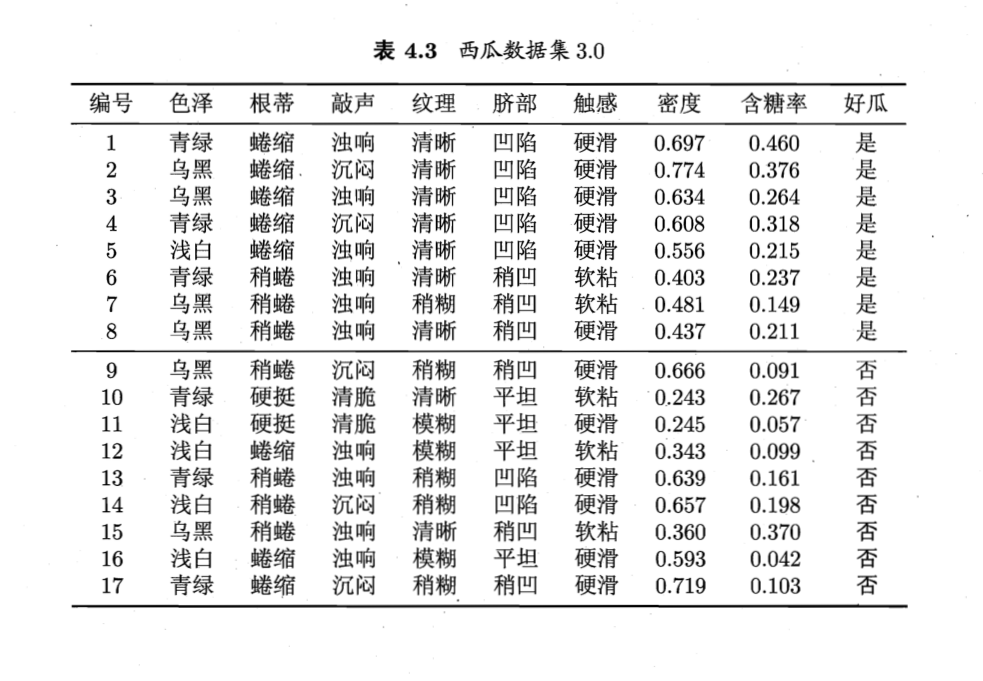代码实现：
import pandas as pd

datasets = pd.read_csv(r'E:\Program Files\PyCharm 2019.2\machinelearning\homework\西瓜3.0.csv', encoding="gbk")
del datasets['编号']
del datasets['好瓜']
return datasets

def NaiveBayes(test):
data = data.values.tolist()
for i in range(len(data)):
if data[i] == 1:
goodMelon.append(data[i])
else:
# 计算p(x|C1)=p1与p(x|C2)=p2
p1 = 1.0;p2 = 1.0
for j in range(len(test)):
x=0.0
for k in range(len(goodMelon)):
if goodMelon[k][j] == test[j]:
x = x + 1.0
p1 = p1 * ((x + 1.0) / (len(goodMelon) + 2.0))  # 拉普拉斯平滑
for j in range(len(test)):
x=0.0
x = x + 1.0
p2 = p2 * ((x + 1.0) / (len(badMelon) + 2.0))  # 拉普拉斯平滑
pc1 = len(goodMelon) / len(data)
pc2 = 1 - pc1
#贝叶斯公式
print('好瓜')
else:
print('坏瓜')

if __name__ == '__main__':
test=['青绿','蜷缩','浊响','清晰','凹陷','硬滑',0.697,0.460]
NaiveBayes(test)


结果：好瓜，分类正确。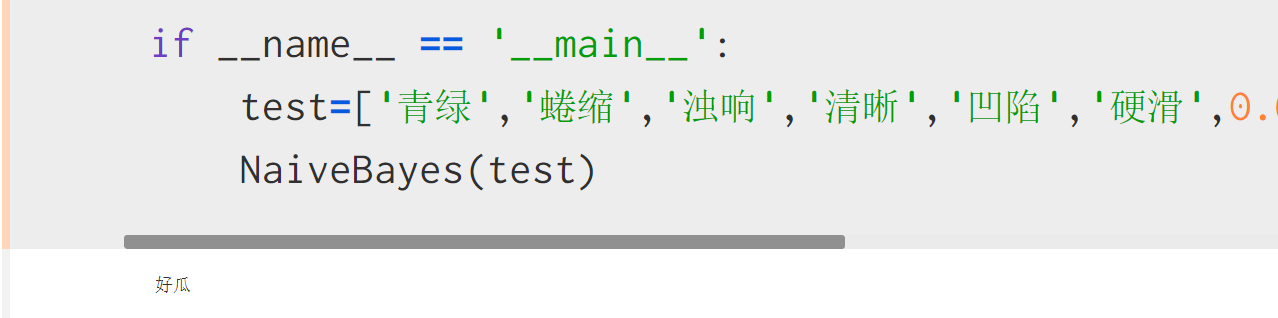展开全文机器学习
• 本内容将介绍 贝叶斯决策论、朴素贝叶斯分类器 和 半朴素贝叶斯分类器
  本内容将介绍 贝叶斯决策论、朴素贝叶斯分类器 和 半朴素贝叶斯分类器。在下一篇中介绍 贝叶斯网络。

贝叶斯分类 是一类分类算法的总称，这类算法均以贝叶斯定理为基础，故统称为贝叶斯分类。

阅读本内容时，需要具备一定的概率论和统计学知识。在 这里 介绍了相关知识点，您可以先简单阅览一下；也可以直接阅读本内容，当遇到不了解的知识点时，再进行了解。

1.1 贝叶斯定理
在概率论与统计学中，贝叶斯定理（Bayes Theorem，也称为贝叶斯法则）描述一个事件的概率，基于可能与该事件相关的条件的先验知识。
贝叶斯定理可以使用以下数学公式描述
$P(A|B) = \frac{P(A)P(B|A)}{P(B)} \tag{1.1}$
对上面公式中的各个概率说明如下：

$P(A|B)$ 是已知 $B$ 发生后 $A$ 的条件概率，也由于得自 $B$ 的取值而被称为 $A$ 的后验概率。

$P(A)$ 是 $A​$ 的先验概率或边缘概率。

$P(B|A)$ 是已知 $A$ 发生后 $B$ 的条件概率，也称为 $B$ 的后验概率和似然概率。

$P(B)$ 是 $B​$ 的先验概率或边缘概率。

按这些术语，贝叶斯定理可表述为
后验概率 = （似然度 * 先验概率） / 标准化常量

也就是说，后验概率与先验概率和似然度的乘积成正比。
另外，有时将 $P(B|A) / P(B)$ 称为标准似然度，贝叶斯定理可表述为
后验概率 = 标准似然度 * 先验概率

1.2 贝叶斯决策论
贝叶斯决策论（Bayesian decision theory）是概率框架下实施决策的基本方法。对分类任务来说，在所有概率都已知的理想情况下，贝叶斯决策论考虑如何基于这些概率和误判损失来选择最优的类别标记。
假设有 $K​$ 种可能的类别标记，即 $\mathcal{Y} = \{ c_1,c_2,\cdots,c_K \}​$，$\lambda_{ij}​$ 表示将一个真实标记为 $c_j​$ 的样本误分类为 $c_i​$ 所产生的损失。基于后验概率 $P(c_i | \mathbf{x})​$ 可获得将样本 $\mathbf{x}​$ 分类为 $c_i​$ 所产生的期望损失，即在样本 $\mathbf{x}​$ 上的“条件风险”
$R(c_i | \mathbf{x}) = \sum_{j=1}^{K} \lambda_{ij} P(c_j | \mathbf{x}) \tag{1.2}$
我们的任务是寻找一个判定准则 $f:\mathcal{X} \rightarrow \mathcal{Y}​$ 以最小化总体风险
$R(f) = \mathbb{E}_{\mathbf{x}} [R(f(\mathbf{x}) \; | \; \mathbf{x})] \tag{1.3}$
显然，对每个样本 $\mathbf{x}​$，若 $f​$ 能最小化条件风险 $R(f(\mathbf{x}) \; | \; \mathbf{x})​$，则总体风险 $R(f)​$ 也将被最小化。这就产生了 贝叶斯判定准则（Bayes decision rule）：为最小化总体风险，只需在每个样本上选择那个能使条件风险 $R(c|\mathbf{x})​$ 最小的类别标记，即
$f^{*}(\mathbf{x}) = \arg\min_{c \in \mathcal{Y}} R(c|\mathbf{x}) \tag{1.4}$
此时，$f^{*}$ 称为 贝叶斯最优分类器（Bayes optimal classifier），与之对应的总体风险 $R(f^*)$ 称为 贝叶斯风险（Bayes risk）。$1-R(f^{*})​$ 反映了分类器所能达到的最好性能，即通过机器学习所能产生的模型精度的理论上限。
具体来说，若目标是最小化分类错误率，则误判损失 $\lambda_{ij}​$ 可写为
$\lambda_{ij} = \left \{ \begin{array}{cc} 0, & if(i=j) \\ 1, & otherwise \end{array} \right. \tag{1.5}$
此时条件风险
$R(c|\mathbf{x}) = 1 - P(c|\mathbf{x}) \tag{1.6}$
于是，最小化分类错误率的贝叶斯最优分类器 为
$f^{*}(\mathbf{x}) = \arg \max_{c\in\mathcal{Y}} P(c | \mathbf{x}) \tag{1.7}$
即对每个样本 $\mathbf{x}$，选择能使后验概率 $P(c|\mathbf{x})​$ 最大的类别标记。
不难看出，欲使用贝叶斯判定准则来最小化决策风险，首先要获得 后验概率 $P(c|\mathbf{x})​$。然而，在现实任务中这通常难以直接获得。从这个角度来看，机器学习所要实现的是基于有限的训练样本集尽可能准确地估计出后验概率 $P(c|\mathbf{x})​$。大体来说，主要有两种策略：给定 $\mathbf{x}​$，可通过直接建模 $P(c|\mathbf{x})​$ 来预测 $c​$，这样得到的是“判别式模型” （discriminative models）；也可先对联合概率分布 $P(\mathbf{x},c)​$ 建模，然后再由此获得 $P(c|\mathbf{x})​$，这样得到的是“生成式模型”（generative models）。比如，决策树、支持向量机等，都可归入判别式模型的范畴。对生成式模型来说，必然考虑
$P(c|\mathbf{x}) = \frac{P(\mathbf{x},c)}{P(\mathbf{x})} \tag{1.8}$
基于贝叶斯定理，$P(c|\mathbf{x})​$ 可写为
$P(c|\mathbf{x}) = \frac{P(c) P(\mathbf{x}|c)}{P(\mathbf{x})} \tag{1.9}$
其中，$P(c)$ 是 类先验概率；$P(\mathbf{x}|c)$ 是样本 $\mathbf{x}$ 相对于类标记 $c$ 的 类条件概率，或称为 似然（likelihood）；$P(\mathbf{x})$ 是用于归一化的“证据”因子。对给定样本 $\mathbf{x}$，证据因子 $P(\mathbf{x})$ 与类标记无关，因此估计 $P(c|\mathbf{x})$ 的问题就转化为基于训练集 $D$ 来估计先验 $P(c)$ 和似然 $P(\mathbf{x}|c)$。

1.3 估计先验 $P(c)$ 和 似然 $P(x_i|c)$
1.3.1 估计先验 $P(c)$
类先验概率 $P(c)$ 表达了样本空间中各类样本所占的比例，根据大数定理，当训练集包含充足的独立同分布样本时，$P(c)​$ 可通过各类样本出现的频率来进行估计。
令 $D_c$ 表示训练集 $D$ 中第 $c$ 类样本组成的集合，若有充足的独立同分布样本，则可容易地估计出类先验概
$P(c) = \frac{|D_c|}{|D|} \tag{1.10}$

1.3.2 估计似然 $P(x_i|c)$
（1）$x_i$ 为离散属性
如果 $x_i$ 为离散属性，采用和估计先验 $P(c)​$ 相同的方法，使用频率来进行估计。
令 $D_{c,x_i}$ 表示 $D_c$ 中第 $i$ 个属性上取值为 $x_i$ 的样本组成的集合，则条件概率 $P(x_i | c)$ 可估计为
$P(x_i | c) = \frac{|D_{c,x_i}|}{|D_c|} \tag{1.11}$
（2）$x_i$ 为连续属性
如果 $x_i$ 为连续属性，估计似然 $P(x_i|c)$ 的一种常用策略是先假定其具有某种确定的概率分布形式，再基于训练样本对概率分布的参数进行估计。假设 $P(x_i|c)$ 具有确定的形式并且被参数 $\theta_c$ 唯一确定，则我们的任务就是利用训练集 $D$ 估计参数 $\theta_c$。为明确起见，我们将 $P(x_i|c)$ 记为 $P(x_i | \theta_c)$。
下面我们将介绍使用源自频率学派的 极大似然估计（MLE）来估计参数 $\theta_c​$。如果您还不了解极大似然估计，可以参阅 这里。
令 $D_c​$ 表示训练集 $D​$ 中第 $c​$ 类样本组成的集合，假设这些样本是独立同分布的，则参数 $\theta_c​$ 对于数据集 $D_c​$ 的似然是
$L(D_c|\theta_c) = \prod_{x_i \in D_c} P(x_i|\theta_c) \tag{1.12}$
对 $\theta_c​$ 进行极大似然估计，就是去寻找能最大化似然 $P(D_c|\theta_c)​$ 的参数值 $\hat \theta_c​$ 。直观上看，极大似然估计是试图在 $\theta_c​$ 所有可能的值中，找到一个能使数据出现的“可能性”最大的值。
式（1.12）中的连乘操作易造成下溢，通常使用对数似然（log-likelihood）
\begin{aligned} L(\theta_c) &= \ln P(D_c | \theta_c) \\\\ &= \sum_{x_i \in D_c} \ln P(x_i | \theta_c) \end{aligned} \tag{1.13}
此时参数 $\theta_c​$ 的极大似然估计 $\hat \theta_c​$ 为
$\hat \theta_c = \arg\max_{\theta_c} L(\theta_c) \tag{1.14}$
假设概率密度函数 $p(x_i|c) \thicksim \mathcal{N}(\mu_{c,i}, \; \sigma_{c,i}^{2}) ​$，其中 $\mu_{c,i}​$ 和 $\sigma_{c,i}^{2}​$ 分别是第 $c​$ 类样本在第 $i​$ 个属性上取值的均值和方差，则参数 $\mu_{c,i}​$ 和 $\sigma_{c,i}^{2}​$ 的极大似然估计为（详细求解过程，可以参阅 这里）
$\hat{\mu}_{c,i} = \frac{1}{|D_c|} \sum_{x_i \in D_c} x_i \tag{1.15}$
$\hat{\sigma}_{c,i}^{2} = \frac{1}{|D_c|} \sum_{x_i \in D_c}(x_i - \hat{\mu}_{c,i})^2 \tag{1.16}$
在求得 $\mu_{c,i}​$ 和 $\sigma_{c,i}^{2}​$ 之后，我们可得到
$p(x_i|c) = \frac{1}{\sqrt{2\pi} \hat{\sigma}_{c,i}} \exp \left( -\frac{(x_i-\hat{\mu}_{c,i})^2}{2 \hat{\sigma}_{c,i}^{2}} \right) \tag{1.17}$
也就是说，通过极大似然法得到的正态分布均值就是样本均值，方差就是 $(x_i - \hat{\mu}_{c,i})^2​$ 的均值，这显然是一个符合直觉的结果。
需注意的是，这种参数化的方法虽能使类条件概率估计变得相对简单，但估计结果的准确性严重依赖于所假设的概率分布形式是否符合潜在的真实数据分布。在现实应用中，欲做出能较好地接近潜在真实分布的假设，往往需在一定程度上利用关于应用任务本身的经验知识，否则若仅凭“猜测”来假设概率分布形式，很可能产生误导性的结果。

1.4 朴素贝叶斯分类器
1.4.1 朴素贝叶斯分类器的定义
对似然 $P(\mathbf{x}|c)$ 来说，由于它涉及关于 $\mathbf{x}$ 所有属性的联合概率，直接根据样本出现的频率来估计将会出遇到严重的困难。例如，假设样本的 $n$ 个属性都是二值的，则样本空间将有 $2^n$  种可能的取值，在现实应用中，这个值往往远大于训练样本数 $m$，也就是说，很多样本取值在训练集中根本没有出现，直接使用频率来估计 $P(\mathbf{x}|c)​$ 显然不可行，因为“未被观测到”与“出现概率为零”通常是不同的。
为避开这个障碍，朴素贝叶斯分类器（Naive Bayes Classifier）采用了 “属性条件独立性假设”：对已知类别，假设所有属性相互独立。换言之，假设每个属性独立地对分类结果发生影响。
基于属性条件独立性假设，式（1.9）可重写为
$P(c|\mathbf{x}) =\frac{P(c) P(\mathbf{x}|c)}{P(\mathbf{x})} =\frac{P(c)}{P(\mathbf{x})} \prod_{i=1}^{n}P(x_i | c) \tag{1.18}$
其中，$n$ 为属性数目，$x_i$ 为 $\mathbf{x}$ 在第 $i$ 个属性上的取值。
由于对所有类别来说 $P(\mathbf{x})​$ 相同，因此基于式（1.7）的贝叶斯判定准则有
$h_{nb}(\mathbf{x}) = \arg \max_{c\in \mathcal{Y}} P(c) \prod_{i=1}^{n}P(x_i | c) \tag{1.19}$
这就是 朴素贝叶斯分类器的表达式。
显然，朴素贝叶斯分类器的训练过程就是基于训练集 $D​$ 来估计类先验概率 $P(c)​$，并为每个属性估计条件概率 $P(x_i |c)​$。

1.4.2 示例
下面采用西瓜书中的例子进行说明，表 1.1 是西瓜数据集 3.0。
表 1.1 西瓜数据集 3.0

编号
色泽
根蒂
敲声
纹理
脐部
触感
密度
含糖率
好瓜

1
青绿
蜷缩
浊响
清晰
凹陷
硬滑
0.697
0.460
是

2
乌黑
蜷缩
沉闷
清晰
凹陷
硬滑
0.774
0.376
是

3
乌黑
蜷缩
浊响
清晰
凹陷
硬滑
0.634
0.264
是

4
青绿
蜷缩
沉闷
清晰
凹陷
硬滑
0.608
0.318
是

5
浅白
蜷缩
浊响
清晰
凹陷
硬滑
0.556
0.215
是

6
青绿
稍蜷
浊响
清晰
稍凹
软粘
0.403
0.237
是

7
乌黑
稍蜷
浊响
稍糊
稍凹
软粘
0.481
0.149
是

8
乌黑
稍蜷
浊响
清晰
稍凹
硬滑
0.437
0.211
是

9
乌黑
稍蜷
沉闷
稍糊
稍凹
硬滑
0.666
0.091
否

10
青绿
硬挺
清脆
清晰
平坦
软粘
0.243
0.267
否

11
浅白
硬挺
清脆
模糊
平坦
硬滑
0.245
0.057
否

12
浅白
蜷缩
浊响
模糊
平坦
软粘
0.343
0.099
否

13
青绿
稍蜷
浊响
稍糊
凹陷
硬滑
0.639
0.161
否

14
浅白
稍蜷
沉闷
稍糊
凹陷
硬滑
0.657
0.198
否

15
乌黑
稍蜷
浊响
清晰
稍凹
软粘
0.360
0.370
否

16
浅白
蜷缩
浊响
模糊
平坦
硬滑
0.593
0.042
否

17
青绿
蜷缩
沉闷
稍糊
稍凹
硬滑
0.719
0.103
否

现在我们需要根据西瓜数据集 3.0 的数据要对下面的测试例进行分类：

编号
色泽
根蒂
敲声
纹理
脐部
触感
密度
含糖率
好瓜

测1
青绿
蜷缩
浊响
清晰
凹陷
硬滑
0.697
0.460

具体计算过程如下：

估计类先验概率 $P(c)$
$P(好瓜=是) = \frac{8}{17} \approx 0.471$
$P(好瓜=否) = \frac{9}{17} \approx 0.529$

估计每个属性的条件概率 $P(x_i | c)$
(1) 离散属性
$P_{青绿|是} = P(色泽=青绿 | 好瓜=是) = \frac{3}{8} = 0.375$
$P_{青绿|否} = P(色泽=青绿 | 好瓜=否) = \frac{3}{9} \approx 0.333$
$P_{蜷缩|是} = P(根蒂=蜷缩 | 好瓜=是) = \frac{5}{8} = 0.625$
$P_{蜷缩|否} = P(根蒂=蜷缩 | 好瓜=否) = \frac{3}{9} \approx 0.333$
$P_{浊响|是} = P(敲声=浊响 | 好瓜=是) = \frac{6}{8} = 0.750$
$P_{浊响|否} = P(敲声=浊响 | 好瓜=否) = \frac{4}{9} \approx 0.444$
$P_{清晰|是} = P(纹理=清晰 | 好瓜=是) = \frac{7}{8} = 0.875$
$P_{清晰|否} = P(纹理=清晰 | 好瓜=否) = \frac{2}{9} \approx 0.222$
$P_{凹陷|是} = P(脐部=凹陷 | 好瓜=是) = \frac{6}{8} = 0.750$
$P_{凹陷|否} = P(脐部=凹陷 | 好瓜=否) = \frac{2}{9} \approx 0.222$
$P_{硬滑|是} = P(触感=硬滑 | 好瓜=是) = \frac{6}{8} = 0.750$
$P_{硬滑|否} = P(触感=硬滑 | 好瓜=否) = \frac{6}{9} \approx 0.667$
(2) 连续属性
针对属性“密度”和“含糖率”，我们都假设其服从高斯分布，然后求其均值和方差。
“密度”属性：$\mu_{密度|是} \approx 0.574$ 和 $\sigma_{密度|是}^{2}\approx 0.129^2$；$\mu_{密度|否} \approx 0.496$ 和 $\sigma_{密度|否}^{2}\approx 0.195^2$。
$p_{密度:0.697|是} = p(密度=0.697 | 好瓜=是) = \frac{1}{\sqrt{2\pi}\cdot0.129} \exp \left( -\frac{(0.697-0.574)^2}{2\cdot0.129^2} \right) \approx 1.959$
$p_{密度:0.697|否} = p(密度=0.697 | 好瓜=否) = \frac{1}{\sqrt{2\pi}\cdot0.195} \exp \left( -\frac{(0.697-0.496)^2}{2\cdot0.195^2} \right) \approx 1.203$
”含糖量“属性：$\mu_{含糖率|是} \approx 0.279$ 和 $\sigma_{含糖率|是}^{2}\approx 0.101^2$；$\mu_{含糖率|否} \approx 0.154$ 和 $\sigma_{含糖率|否}^{2}\approx 0.108^2$。
$p_{含糖率:0.460|是} = p(含糖率=0.460 | 好瓜=是) = \frac{1}{\sqrt{2\pi}\cdot0.101} \exp \left( -\frac{(0.697-0.279)^2}{2\cdot0.101^2} \right) \approx 0.788$
$p_{含糖率:0.460|否} = p(含糖率=0.460 | 好瓜=否) = \frac{1}{\sqrt{2\pi}\cdot0.108} \exp \left( -\frac{(0.697-0.154)^2}{2\cdot0.108^2} \right) \approx 0.066$

计算概率
$P_{青绿|是} \times P_{蜷缩|是} \times P_{浊响|是} \times P_{清晰|是} \times P_{凹陷|是} \times P_{硬滑|是}\times p_{密度:0.697|是} \\ \times p_{含糖率:0.460|是} \times P(好瓜=是) \approx 0.038$
$P_{青绿|否} \times P_{蜷缩|否} \times P_{浊响|否} \times P_{清晰|否} \times P_{凹陷|否} \times P_{硬滑|否}\times p_{密度:0.697|否} \\ \times p_{含糖率:0.460|否} \times P(好瓜=否) \approx 6.80 \times 10^{-5}$
由于 $0.038 > 6.80 \times 10^{-5}$，因此，朴素贝叶斯分类器将测试样本“测 1”判别为“好瓜”。需注意，在实际应用中，我们通常取对数，将“连乘”转化为“连加”以避免数值下溢。

1.4.3 拉普拉斯修正
针对 离散型属性，如果某个属性值在训练集中没有与某个类同时出现过，则直接基于式（1.11）进行概率估计得到 $P(x_i|c) = 0$；再根据式（1.18）进行连乘计算出的概率值为 $0$。也就是说不管其他属性如何取值，都会因为 $P(x_i|c) = 0$ 导致分类器认为该样本属于类型 $c$ 的概率为 $0​$，这显然是不合理的。
为了避免这个问题的出现，因此在估计概率值时通常要进行 “平滑”（smoothing），常用 “拉普拉斯修正”（Laplacian Correction）。具体来说，令 $N$ 表示训练集 $D$ 中可能的类别数，$N_i$ 表示第 $i$ 个属性可能的取值数量，则式（1.10）和式（1.11）分别修正为
$\hat{P}(c) = \frac{|D_c| + 1}{|D| + N} \tag{1.20}$
$\hat{P}(x_i | c) = \frac{|D_{c,x_i}| + 1}{|D_c| + N_i} \tag{1.21}$
显然，拉普拉斯修正避免了因训练集样本不充分而导致概率估计为零的问题，并且在训练集变大时，修正过程所引入的先验的影响也会逐渐变得可忽略，使得估值渐趋向于实际概率值。

1.4.4 总结
朴素贝叶斯法是基于贝叶斯定理与特征条件独立假设的分类方法。对于给定的训练数据集，首先基于特征条件独立假设，学习输入/输出的联合概率分布；然后基于此模型，对给定的输入 $\mathbf{x}$，利用贝叶斯定理求出后验概率最大的输出 $y$。朴素贝叶斯法实现简单，学习与预测的效率都很高，是一种常用的方法。
在现实任务中，朴素贝叶斯分类器有多种使用方式。例如，如果任务对预测速度要求较高，则对给定训练集，可将朴素贝叶斯分类器涉及的所有概率估值事先计算好并存储起来，这样在进行预测时只需“查表”即可进行判别；如果任务数据更替频繁，则可采用“懒惰学习”（lazy learning）方式，先不进行任何训练，待收到预测请求时再根据当前数据集进行概率估值；如果数据不断增加，则可在现有估值的基础上，仅对新增样本的属性值所涉及的概率估值进行计数修正即可实现增量学习。

1.5 半朴素贝叶斯
为了降低贝叶斯公式（1.9）中估计后验概率 $P(c|\mathbf{x})​$ 的困难，朴素贝叶斯分类器采用了属性条件独立性假设，但在现实任务中这个假设很难成立。于是，人们尝试对属性条件独立性假设进行一定程度的放松，由此产生了一类称为“半朴素贝叶斯分类器”（Semi-naive Bayes Classifiers）的学习方法。
半朴素贝叶斯分类器的基本想法是适当考虑一部分属性间的相互依赖信息，从而既不需要进行完全联合概率计算，又不至于彻底忽略了比较强的属性依赖关系。“独依赖估计”（One-Dependent Estimator，简称 ODE）是半朴素贝叶斯分类器最常用的一种策略。顾名思义，所谓“独依赖”就是假设每个属性在类别之外最多仅依赖于一个其他属性，即
$P(c|\mathbf{x}) \propto P(c) \prod_{i=1}^{n}P(x_i|c,pa_i) \tag{1.22}$
其中 $pa_i​$ 为属性 $x_i​$ 所依赖的属性，称为 $x_i​$ 的父属性。此时，对每个属性 $x_i​$，如果父属性 $pa_i​$ 已知，则可采用类似式（1.21）的办法来估计概率值 $P(x_i | c,pa_i)​$。于是，问题的关键就转化为如何确定每个属性的父属性，不同的做法产生不同的独依赖分类器。
最直接的做法是假设所有属性都依赖于同一个属性，称为“超父”（super-parent），然后通过交叉验证等模型选择方法来确定超父属性，由此形成了 SPODE（Super-Parent ODE）方法。例如，在图 1.1（b）中，$x_1​$ 是超父属性。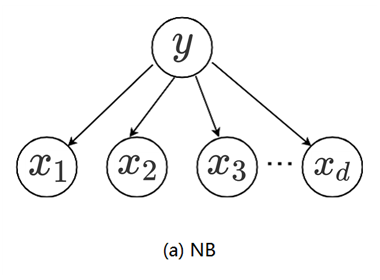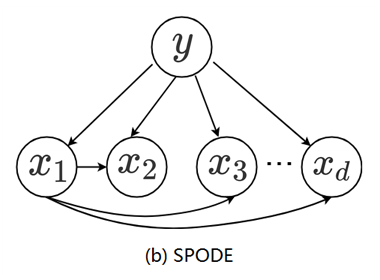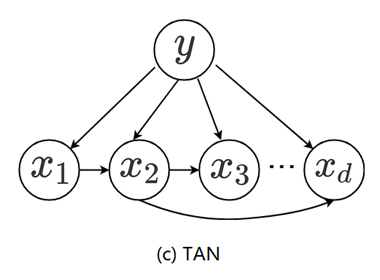图 1.1    朴素贝叶斯与两种半朴素贝叶斯分类器所考虑的属性依赖关系

TAN（Tree Augmented Naive Bayes）则是在最大带权生成树（maximum weighted spanning tree）算法的基础上，通过以下步骤将属性间依赖关系约简为如图 1.1（c）所示的树形结构：

计算任意两个属性之间的条件互信息（conditional mutual information）
$I(x_i,x_j|y) = \sum_{x_i,y_i;c\in\mathcal{Y}} P(x_i,x_j|c) \log \frac{P(x_i,x_j|c)}{P(x_i|c)P(x_j|c)} \tag{1.23}$

以属性为结点构建完全图，任意两个结点之间边的权重设为 $I(x_i,x_j|y)$。

构建此完全图的最大带权生成树，挑选根变量，将边置为有向。

加入类别结点 $y$，增加从 $y​$ 到每个属性的有向边。

容易看出，条件互信息 $I(x_i,x_j|y)$ 刻画了属性 $x_i$ 和 $x_j​$ 在已知类别情况下的相关性，因此，通过最大生成树算法，TAN 实际上仅保留了强相关属性之间的依赖性。
AODE（Averaged One-Dependent Estimator）是一种基于集成学习机制、更为强大的独依赖分类器。与 SPODE 通过模型选择确定超父属性不同，AODE 尝试将每个属性作为超父来构建 SPODE，然后将那些具有足够训练数据支撑的 SPODE 集成起来作为最终结果，即
$P(c|\mathbf{x}) \propto \sum_{i=1, \; |D_{x_i}|\geq m^{'}}^{n} P(c,x_i) \prod_{j=1}^{n}P(x_j | c,x_i) \tag{1.24}$
其中 $D_{x_i}$ 是在第 $i$ 个属性上取值为 $x_i$ 的样本的集合，$m^{'}$ 为阈值常数（一般默认设置为 30）。显然 AODE 需估计  $P(c,x_i)$ 和 $P(x_j|c,x_i)$。类似式（7.20），有
$\hat{P}(c,x_i) = \frac{|D_{c,x_i}|+1}{|D|+N_i} \tag{1.25}$
$\hat{P}(x_j|c,x_i) = \frac{|D_{c,x_i,x_j}|+1}{|D_{c,x_i}|+N_j} \tag{1.26}$
其中 $N_i$ 是第 $i$ 个属性可能的取值数量，$D_{c,x_i}$ 是类别为 $c$ 且在第 $i$ 个属性上取值为 $x_i$ 的样本集合，$D_{c,x_i,x_j}$ 是类别为 $c$ 且在第 $i$ 个和第 $j$ 个属性上取值分别为 $x_i$ 和 $x_j​$ 的样本集合。
不难看出，与朴素贝叶斯分类器类似，AODE 的训练过程也是“计数”，即在训练数据集上对符合条件的样本进行计数的过程。与朴素贝叶斯分类器相似，AODE 无需模型选择，即能通过预计算节省预测时间，也能采取懒惰学习方式在预测时再进行计数，并且易于实现增量学习。
既然将属性条件独立性假设放松为独依赖假设可能获得泛化性能的提升，那么，能否通过考虑属性间的高阶依赖（即对多个属性依赖）来进一步提升泛化性能呢？也就是说，将式（1.22）中的属性 $pa_i​$ 替换为包含 $k​$ 个属性的集合 $\mathbf{pa_i}​$，从而将 ODE 拓展为 $k​$DE。需注意的是，随着 $k​$ 的增加，准确估计概率 $P(x_i |y, \mathbf{pa_i})​$ 所需的训练样本数量将以指数级增加。因此，若训练数据非常充分，泛化性能有可能提升；但在有限样本条件下，则又陷入估计高阶联合概率的泥沼。

参考：
 周志华《机器学习》
 李航《统计学习方法》
 算法杂货铺——分类算法之朴素贝叶斯分类(Naive Bayesian classification)


展开全文贝叶斯分类 贝叶斯定理 贝叶斯决策论
• 朴素贝叶斯分类器 MATLAB 源代码，里面含有使用实例，用的是 UCI 的 mushroom 数据集。 分类器详细介绍见： http://blog.csdn.net/yunduanmuxue/article/details/39693917
• 临近期末考试，简单总结了一下朴素贝叶斯分类器，共3个部分的内容。 朴素贝叶斯分类器的思想 朴素贝叶斯分类器的通用过程 两个具体例子的计算 1 朴素贝叶斯分类器的思想 对于给出的一个待分类项x，求解x出现的...机器学习 西瓜书 NB
• 多项式朴素贝叶斯分类器In Analytics Vidhya, Hackathon, there was a problem statement for text prediction of topic/subject to which class it belongs basis on title and abstract. To solve this question ...
• 机器学习 - 朴素贝叶斯（下） 朴素贝叶斯分类器 朴素贝叶斯 重要假设 特征类型 朴素贝叶斯分类模型 举例 贝叶斯估计 模型特点朴素贝叶斯 拉普拉斯平滑 贝叶斯估计
• 主要为大家详细介绍了python实现朴素贝叶斯分类器，具有一定的参考价值，感兴趣的小伙伴们可以参考一下
• 基于权重的朴素贝叶斯分类器设计与实现基于权重的朴素贝叶斯分类器设计与实现基于权重的朴素贝叶斯分类器设计与实现基于权重的朴素贝叶斯分类器设计与实现基于权重的朴素贝叶斯分类器设计与实现基于权重的朴素贝叶斯...
• 实现朴素贝叶斯分类器算法基本功能，代码有注释，还包括一个垃圾邮件过滤的实例。另外我这次用的是python2.7版，如果用python3的可能需要根据提示修改几个语法（sorted函数的参数）。
• 基于Matlab的朴素贝叶斯分类器，该文件为压缩文件，解压后可用。包含了实验数据。是离散类型的朴素贝叶斯分类器
• C++实现朴素贝叶斯分类器, 代码干净, 整洁, 有示例, 有注释, 具有良好的封装性, 可直接迁移使用
• 朴素贝叶斯分类器，顾名思义，是一种分类算法，且借助了贝叶斯定理。另外，它是一种生成模型（generative model），采用直接对联合概率P(x,c)建模，以获得目标概率值的方法。预备知识 先验概率与后验概率 贝叶斯定理...
• 通过《统计学习方法》第4章了解朴素贝叶斯分类器基本原理，同时，“龙心尘”关于朴素贝叶斯的三篇博文也受益匪浅。博客地址：http://blog.csdn.net/longxinchen_ml/article/details/50597149 本文总结朴素贝叶斯的...
• 本资源利用朴素贝叶斯分类器实现了数据的多分类。文件包括朴素贝叶斯分类器的实现代码，训练数据和测试数据以及对应的类别标签。分类救结果较好，能达到91.25%。...